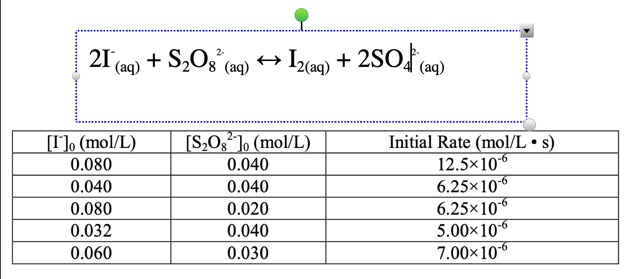## Short Problem

Consider the following reaction conducted at 298K:
SO2 + O3 → SO3 + O2
Notice that's ozone, O3, on the left. Write the rate expression for this reaction in turns of all four components (how you would follow the reaction).
Then, write the rate law for the equation. Determine the reaction order for SO2, and O3. Determine the overall reaction order.
Determine the value of "k" for this reaction (at 298K).
What are the units of k?

For the second problem, with data below, answer the same set of questions. Get the reaction order in each component, the overall reaction order, the value and units of "k."Back
MathJax Font